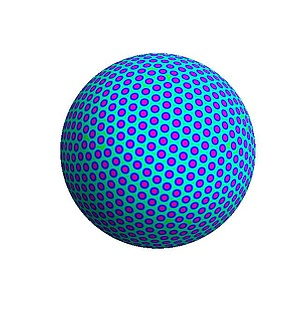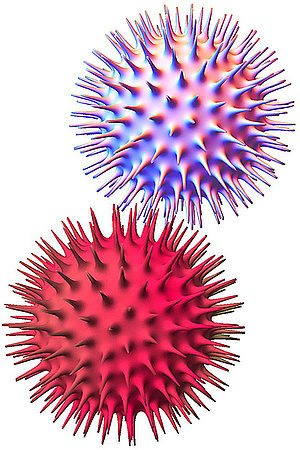# Analysis

The research in analysis at the institute ranges from real and complex analysis, asymptotic analysis, analytic combinatorics, over special functions and differential equations to general topology.The following members of this institute work in analysis.

• Tichy, Robert (Discrepancy theory, Diophantine equations, analytic and algorithmic number theory, Quasi-Monte Carlo methods and applications in mathematical finance)
• Grabner, Peter (Uniform distribution and arithmetic dynamical systems, asymptotic analysis, analytic combinatorics, Fractal Structures in Number Theory)
• Aistleitner, Christoph (probabilistic methods in analysis and number theory, analytic number theory, limit laws in probability)
• Elsholtz, Christian (analytic and combinatorial number theory)
• Ganster, Maximilian (general topology)
• Tomantschger, Kurt (complex analytic methods in partial differential equations, special functions)
• Brauchart, Johann (point distributions and Quasi-Monte Carlo methods  on spheres and manifolds, self organization by local interaction)
• Borda, Bence (discrete and continuous uniform distribution, lattice points in convex bodies, random walks on groups)
• Quesada Herrera, Emily (Analytic number theory, Riemann zeta-function, Fourier uncertainty, binary quadratic forms)
• Rasskin, Ivan (discrete geometry, fractals in Number Theory, Knot Theory)
• Wibmer Michael (differential equations, differential Galois theory)

PhD students:

Michael Henry

• Former members:
• Berglez, Peter (complex analytic methods in partial differential equations, special functions)
• Ujué Etayo (uniform point distributions on spheres and manifolds, quadrature rules, asymptotic analysis, real complexity theory)
• Kusner, Woden (discrete and optimal geometry)
• Ferizovic, Damir
• fractal geometry, topology of fractals, discrete structures, uniform distribution,digital expansions, combinatorics on words)
• (Discrepancy Theory, Potential Theory, and optimal point distributions)

Several externally funded research projects were devoted to related subjects in recent years. Currently, the Special Research Area (SFB) "Quasi-Monte Carlo Methods: Theory and Applications" combines forces of our group with other research groups in Linz, Salzburg, and Vienna.